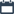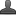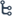## Thursday, October 29, 2020

•October 29, 2020
••, , ,
•No comments

## Important questions for 10th class Maths chapter-8 Similar triangles,Mathematics questionnaire-x class

8. SIMILAR TRIANGLES

Hi friends and my dear students! In this post, I have covered Important questions for 10th class maths chapter-8 Similar triangles, Chapter wise previous year Questions. After Reading  Please do share it with your friends. Learn maths for All classes here

##

PRIORITY-I

1 MARK QUESTION

1. What are similar triangles?

2. What are similar polygons?

3. State THALES theorem?

4. Write properties of similar triangles

5. Is similarity of triangles different from similarity of polygons? Why?

6. Seethe said that “square and rhombus are similar figures” do you accept her statement? Justify your answer?

7. State the converse of the Basic proportionality theorem?

8. State AAA similarity criterion ?

9. State SSS similarity criterion ?

10. State SAS similarity criterion ?

11. State Pythagoras theorem?

12. State Converse of Pythagoras Theorem?

13. If the sides of a triangle are 3 cm, 4 cm and 6 cm long, determine whether the triangle is a right-angled triangle.?

2MARKS QUESTIONS:

14. In ΔABC,DE // BC and AD/BD=3/4, AC = 5.6 . Find AE

15. In ΔABC,LM //AB, AL = x-3, AC= 2x, BM = x-2, BC = 2x+3 find the value of x?

16. The perimeters of two similar triangles are 30cm and 20cm respectively. If one side of the first triangle is 12cm determine the corresponding side of the second triangle?

17. In if DE || AB, AD=8x+9, CD=x+3, BE=3x+4 and CE=x then find the value of ‘x’.

18. Give two different examples of i. similar figures ii. non similar figures iii. Congruent figures

19. In a trapezium ABCD, AB|| DC, Eand F are points on non parallel sides AD and BC respectively such EF||AB, then show that AE/ED=BF/FG

20. Prove that a line drawn through the midpoint of one side of a triangle, parallel to another side bisects third side?

21. Prove that a line joining the midpoints of any two sides of a triangle is parallel to the third side?

22. A person 1.65m tall casts 1,8m shadow. At the same instance, a lamp-post casts a shadow of 5.4m. Find the height of the lamp-post?

23. A man sees the top of a tower in a mirror which is at a distance of 87.6m from the tower. The mirror is on the ground facing upwards. The man is away from the mirror and his height is 1.5m. How tall is the tower?

24. A girl of height 90cm is walking away from the base of a lamppost at a speed of 1.2m/sec. If the lamp post is 3.6m above the ground, find the length of her shadow after 4 seconds?

25. A flag pole 4m tall casts a 6m shadow. At the same time, a nearby building casts shadow of 24m, how tall is the building?

26. ΔABC ΔDEF and their areas are 64cm2 and 121cm2 respectively. If EF = 15.4cm, then find BC?

27. Prove that the ratio of if the areas of two similar triangles are equal to the square of the ratio of their corresponding medians?

28. ΔABC ΔDEF.BC = 3cm, EF = 4cm, and area of ΔABC = 54cm2 , determine the area of ΔDEF

29. The areas of two similar triangles are 81cm2 and 49cm2 respectively. If the altitude of the bigger triangle is 4.5cm. find the corresponding altitude of the smaller triangle?

30. A ladder 25m long reaches a window of the building 20m above the ground. Determine the distance of the foot of the ladder from the building?

31. A ladder 15m long reaches a window of the building 9m above the ground on one side of a street . Keeping is foot at the same point , the ladder is turned to other side of the street to reach a window 12m high. Find the width of the street?

32. Hypotenuse of a right triangle is 6m more than twice of it’s the shortest side. If the third side is 2m less than the hypotenuse, find the sides of triangle?

33. ABC is an isosceles right triangle right angled at C. Prove that AB2 = 2AC2

34. A wire attached to vertical pole of height 18m is 24m long and has a stake attached to the other end. How far from the base of the pole should the stake be driven so that the wire will be taut.?

35. Two poles of heights 6m and 11m stand on a plane ground. If the distance between the feet of the poles is 12m , find the distance between their tops.?

Also Check

## Important questions for 10th class Maths chapter-7 Coordinate Geometry

4 MARKS QUESTIONS:

1. State and prove THALES theorem?

2. State and prove converse of THALES theorem.

3. Equilateral triangles are drawn on the three sides of right angled triangle show that the area of the triangle on the hypotenuse is equal to the sum of the areas of triangles on the other two sides.

4. Draw a line segment of length 7.2cm, and divide it in the ratio 5:3. Measure the two parts

5. Construct a triangle shadow similar to the given triangle ΔABC, with its sides equal to 5/3 of corresponding sides of ΔABC?

6. Construct a triangle of sides 4cm, 5cm, 6cm. Then Construct a triangle similar to it, whose sides are 2/3 of corresponding sides of first triangle.

7. Construct an isosceles triangle whose base is 8cm, and altitude is 4cm. Then draw another triangle whose sides are 11/2of corresponding sides of isosceles triangle?

8. Prove that the ratios of the areas of two similar triangles is equal to the ratios of the squares of their corresponding sides

9. State and prove PYTHAGORAS theorem?

10. State and prove converse of PYTHAGORAS theorem?

11. ABC is a right triangle right angle at C. Let BC = a, CA = b, AB = c and p be the length of perpendicular from C on AB. Prove that (i) pc = ab ii.1/P2=1/a2+1/b2?

12. Prove that the sum of the squares of the sides of a rhombus is equal to the sum of the squares of its diagonals?

13. O’ is any point inside a rectangle ABCD. Prove that OB2 + OD2 = OA2 + OC2?

PRIORITY II:

1 MARK QUESTIONS:

1. If the attitude of two similar triangles are in the ratio 2:3 what is ration of their areas

2. If ABC and DEF are two triangles such that AB/DE= BC/EF=CA/FD=3/4 then write ar (∆ABC):ar(∆DEF)

3. Write two examples for similar figures from your daily life

4. What mathematical concept is observed when your photo is enlarged

5. If ABC is a isosceles triangle in which ∟c=900 if AC=6cm then find AB

6. If I DEF are similar such that 2AB=DE and BC=8 Then find EF

7. When do we say two triangles are similar?

8. In what way similarity of triangles is different from similarity of polygons

9. Define regular polygon

10. Give an example from your daily life where scale factor is used

12. Give an example of Pythagorean triplet

13. If the ratio of perimeters of two similar triangles is 4:25 find the ratio of their area

14. Write the relation between the areas and the sides of similar triangles

2 MARKS QUESTIONS:

1. The area of two similar triangles are 160cm2 and 121cm2 respectively if the longest sides of larger triangle is 26cm what is the length of corresponding similar triangle

2. If ABC is equilateral triangle such that AD ┴ BC then find the value of AD2

3. Verify 4cm, 6cm and 8cm from a Pythagoras triplet or not

4. Write the relation between the sides and diagonals of a rhombus?

Ø 4 MARKS QUESTIONS:

1. AB,CD,PQ are perpendicular to BD, AB=x, CD=y and PQ=z prove that 1/x+1/y=1/z

2. In an equilateral triangle ABC, D is a point on side BC such that BD=1/3BC prove that 9AD2=7AB2

3. BL and CM are medians of ΔABC right angle at A. prove that 4(BL2 + CM2 ) = 5BC2?

4. Prove that three times the square of any side of an equilateral triangle is equal to four times the square of the altitude?